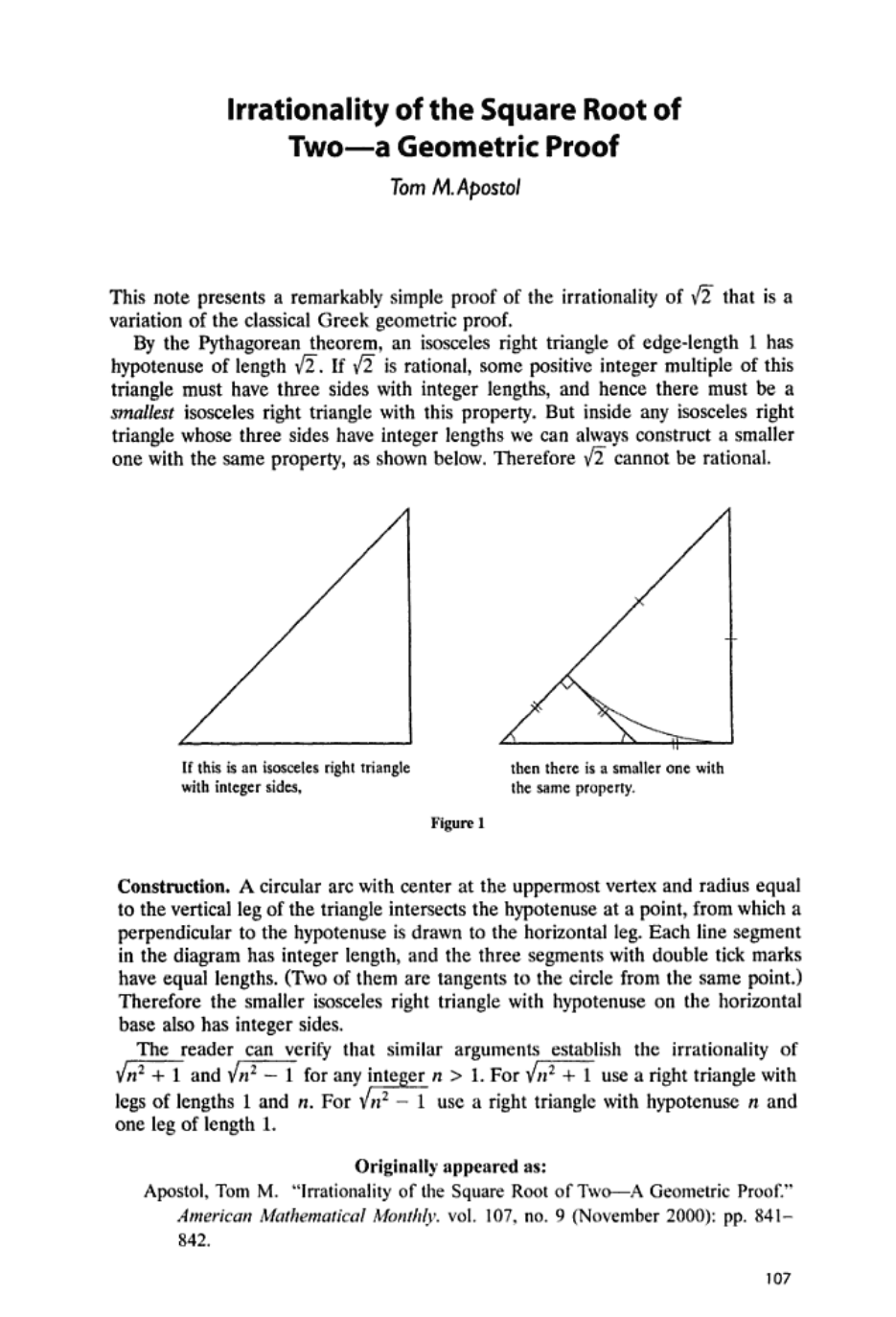prove that 2^1/3 is irrational
Tom Mike Apostol is an American analytic number theorist and profes...We can keep drawing smaller and smaller isosceles right triangles until the area of the triangle drops below $\frac{1}{2}$. In that case we have reached a contradiction and $\sqrt 2$ cannot be a rational number. ![](http://i.imgur.com/McM0Z6d.jpg?1) prove that 2^1/3 is irrational To see the classical Greek Geometric proof [click here](https://en.wikipedia.org/wiki/Square_root_of_2#Geometric_proof). Tom Mike Apostol is an American analytic number theorist and professor at the California Institute of Technology. ![](http://dlmf.nist.gov/about/bio/photo_apostol.jpg) John Conway and Richard Guy actually came up with a similar proof but with a paper folding interpretation. They started with a square paper with a side of length 12 inches. ![](http://i.imgur.com/NjgxLm6.png?1) From the Pythagoras theorem if the length of the side is 12 inches the length of the diagonal is $12\sqrt 2= 16.97056...$ inches. So $\sqrt 2$ is very close to $\frac{17}{12}$ inches. Conway and Guy showed that $\sqrt 2$ cannot be equal to any such fraction. To get there let's suppose that $\sqrt 2 = \frac{17}{12}$, which means the diagonal of a 12 inch square would be exactly 17 inches. Now if we fold AD' onto the diagonal AC to get AD, then CDE is the same shape as ABC (both are isosceles right triangles) with whole number sides: CD = 17-12 = 5 CE = 12 -5 = 7 Finally it is not difficult to see that if we divide every side of the triangle CDE by 5, the hypotenuse has length $\sqrt 2=\frac{7}{5}$, which is an even simpler fraction than $\frac{17}{12}$ (a contradiction!).